Technical Article

# The Variable: A Valuable Object in Sequential VHDL

February 14, 2018 by Dr. Steve Arar

## This article will discuss the important features of variables in VHDL.

This article will discuss the important features of variables in VHDL.

The previous article in this series discussed that sequential statements allow us to describe a digital system in a more intuitive way. Variables are useful objects that can further facilitate the behavioral description of a circuit. This article will discuss the important features of variables. Several examples will be discussed to clarify the differences between variables and signals. Let’s first review VHDL signals.

### Multiple Assignments to a Signal

VHDL uses signals to represent the circuit interconnects or wires. For example, consider the circuit in Figure 1.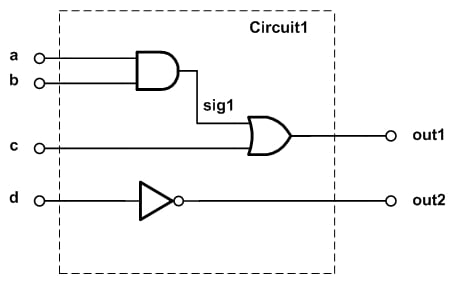##### Figure 1.

1	architecture Behavioral of circuit1 is
2		signal sig1: std_logic;
3	begin
4		sig1 <= ( a and b );
5		out1 <= ( sig1 or c );
6		out2 <= (not d);

7	end Behavioral;

As you can see, a signal has a clear mapping into hardware: it becomes a (group of) wire(s). Does it make sense to have multiple assignments to a signal? For example, consider the following code section:

sig1 <= ( a and b );
sig1 <= (c or d);

If these two assignments are in the concurrent part of the code, then they are executed simultaneously. We can consider the equivalent hardware of the above code as shown in Figure 2.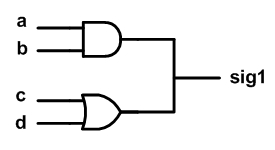##### Figure 2.

Figure 2 suggests that multiple assignments to a signal in the concurrent part of the code is not a good idea because there can be a conflict between these assignments. For example, if A=C=0 and B=D=1, the first line would assign sig1 = (0 and 1) =0, while the second would attempt to assign sig1 = (0 or 1) = 1. That’s why, in the concurrent part of the code, VHDL doesn’t allow multiple assignments to a signal. What if these two assignments were in the sequential part of the code? A compiler may accept multiple assignments inside a process but, even in this case, only the last assignment will survive and the previous ones will be ignored. To explain this, note that a process can be thought of as a black box whose inner operation may be given by some abstract behaviour description. This description uses sequential statements. The connection between the process black box and the outside world is achieved through the signals. The process may read the value of these signals or assign a value to them. So VHDL uses signals to connect the sequential part of the code to the concurrent domain. Since a signal is connected to the concurrent domain of the code, it doesn’t make sense to assign multiple values to the same signal. That’s why, when facing multiple assignments to a signal, VHDL considers only the last assignment as the valid assignment.

### Updating the Value of a Signal

The black box interpretation of a process reveals another important property of a signal assignment inside a process: When we assign a value to a signal inside a process, the new value of the signal won’t be available immediately. The value of the signal will be updated only after the conclusion of the current process run. The following example further clarifies this point. This example uses the VHDL “if” statements. Please note that we’ll see more examples of this statement in future articles; however, since it is similar to the conditional structures of other programming languages, the following code should be readily understood. You can find a brief description of this statement in a previous article.

Example: Write the VHDL code for a counter which counts from 0 to 5.

One possible VHDL description is given below:

1	library IEEE;
2	use IEEE.STD_LOGIC_1164.ALL;

3	entity SigCounter is
4	    Port ( clk : in  STD_LOGIC;
5		   out1: out integer range 0 to 5);
6	end SigCounter;

7	architecture Behavioral of SigCounter is

8		signal sig1 : integer range 0 to 6;

9	begin

10		process(clk)
11		begin
12		if (clk'event and clk='1') then
13			sig1 <= sig1+1;
14			if (sig1=6) then
15				sig1 <= 0;
16			end if;
17		end if;
18		out1 <= sig1;
19		end process;

20	end Behavioral;

In this example, sig1 is defined as a signal of type integer in the declarative part of the architecture. With each rising edge of clk, the value of the signal sig1 will increase by one. When sig1 reaches 6, the condition of the “if” statement in line 14 will be evaluated as true and sig1 will take the value zero. So it seems that sig1, whose value is eventually passed to the output port out1, will always take the values in the range 0 to 5. In other words, it seems that the “if” statement of line 14 will never let sig1 take the value 6. Let’s examine the operation of the code more closely.

Assume that a previous run of the process sets sig1 to 5. With the next rising edge of clk, the statements inside the “if” statement of line 12 will be executed. Line 13 will add one to the current value of sig1, which is 5, and assign the result to sig1. Hence, the new value of sig1 will be 6; however, we should note that the value of the signal sig1 will be updated only after the conclusion of the current process run. As a result, in this run of the process, the condition of the “if” statement in line 14 will be evaluated as false and the corresponding “then” branch will be bypassed. Reaching the end of the process body, the value of sig1 will be updated to 6. While we intended sig1 to be in the range 0 to 5, it can take the value 6!

Similarly, at the next rising edge of clk, line 13 will assign 7 to sig1. However, the signal value update will be postponed until we reach the end of the process body. In this run of the process, the condition of the “if” statement in line 14 returns true and, hence, line 15 will set sig1 to zero. As you see, in this run of the process, there are two assignments to the same signal. Based on the discussion of the previous section, only the last assignment will take effect, i.e. the new value of sig1 will be zero. Reaching the end of this process run, sig1 will take this new value. As you see, sig1 will take the values in the range from 0 to 6 rather than from 0 to 5! You can verify this in the following ISE simulation of the code.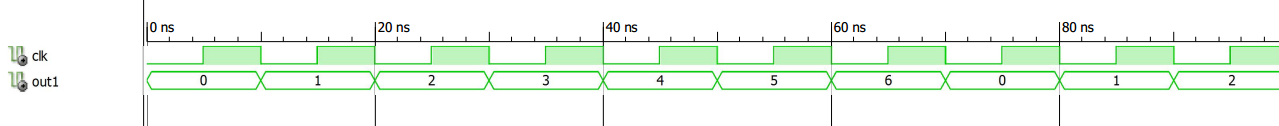##### Figure 3.

Hence, when using signals inside a process, we should note that the new value of a signal will be available at the end of the current run of the process. Not paying attention to this property is a common source of mistake particularly for those who are new to VHDL.

To summarize our discussion so far, a signal models the circuit interconnections. If we assign multiple values to a signal inside a process, only the last assignment will be considered. Moreover, the assigned value will be available at the end of the process run and the updates are not immediate.

### Variable: Another Useful VHDL Object

As discussed in a previous article, sequential statements allow us to have an algorithmic description of a circuit. The code of such descriptions is somehow similar to the code written by a computer programming language. In computer programming, “variables” are used to store information to be referenced and used by programs. With variables, we can more easily describe an algorithm when writing a computer program. That’s why, in addition to signals, VHDL allows us to use variables inside a process. While both signals and variables can be used to represent a value, they have several differences. A variable is not necessarily mapped into a single interconnection. Besides, we can assign several values to a variable and the new value update is immediate. In the rest of the article, we will explain these properties in more detail.

Before proceeding, note that variables can be declared only in a sequential unit such as a process (the only exception is a “shared” variable which is not discussed in this article). To get more comfortable with VHDL variables, consider the following code segment which defines variable var1.

1	process(clk)
2		variable var1 : integer range 0 to 5;
3	begin
4	var1 := 3;
5	...
6	end process;

Similar to a signal, a variable can be of any data type (see the previous articles in this series to learn more about different data types). However, variables are local to a process. They are used to store the intermediate values and cannot be accessed outside of the process. Moreover, as shown by line 4 of the above code, the assignment to a variable uses the “:=” notation, whereas, the signal assignment uses “<=”.

### Multiple Assignments to a Variable

Consider the following code. In this case, a variable, var1, of type std_logic is defined. Then in lines 12, 13, and 14, three values are assigned to this variable.

1	library IEEE;
2	use IEEE.STD_LOGIC_1164.ALL;

3	entity VarTest1 is
4	    Port ( in1, in2, in3 : in  STD_LOGIC;
5                  out1 : out  STD_LOGIC);
6	end VarTest1;

7	architecture Behavioral of VarTest1 is

8	begin

9		process(in1, in2, in3)
10		variable var1: std_logic;
11		begin
12			var1 := in1;
13			var1 := (var1 and in2);
14			var1 := (var1 or in3);
15			out1 <= var1;
16		end process;

17	end Behavioral;

Figure 4 shows the RTL schematic of the above code which is generated by Xilinx ISE.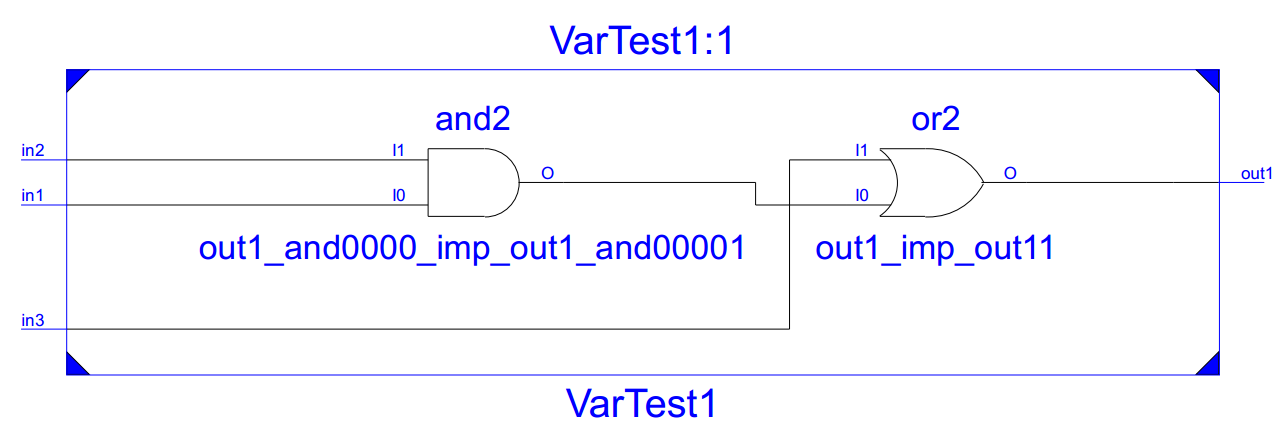##### Figure 4.

It’s easy to verify that the produced schematic matches the behavior described in the process; however, this example shows that mapping variables into the hardware is somehow more complicated than that of signals. This is due to the fact that the sequential statements describe the behavior of a circuit.  As you can see, in this example, each variable assignment operation of lines 13 and 14 have created a different wire though both of these two assignments use the same variable name, i.e. var1.

### Updating the Value of a Variable

Variables are updated immediately. To examine this, we’ll modify the code of the above counter and use a variable instead of a signal. The code is given below:

1	library IEEE;
2	use IEEE.STD_LOGIC_1164.ALL;

3	entity VarCounter is
4	    Port ( clk : in  STD_LOGIC;
5		   out1: out integer range 0 to 5);
6	end VarCounter;

7	architecture Behavioral of VarCounter is

8	begin

9		process(clk)
10		variable var1 : integer range 0 to 6;
11		begin
12		if (clk'event and clk='1') then
13			var1 := var1+1;
14			if (var1=6) then
15			    var1 := 0;
16			end if;
17		end if;
18		out1 <= var1;
19		end process;

20	end Behavioral;

Since the new value of a variable is immediately available, the output will be in the range 0 to 5. This is shown in the following ISE simulation result.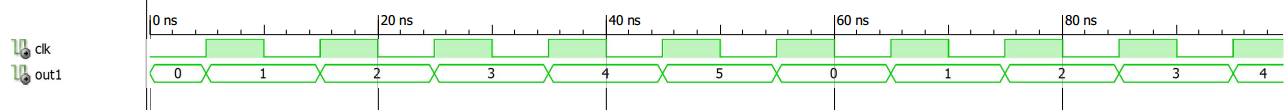### Summary

• A signal models the circuit interconnections. If we assign multiple values to a signal inside a process, only the last assignment will be considered. Moreover, the assigned value will be available at the end of the current process run and the updates are not immediate.
• A single variable can produce several circuit interconnections.
• We can assign multiple values to the same variable and the assigned new values will take effect immediately.
• Similar to a signal, a variable can be of any data type.
• Variables are local to a process. They are used to store the intermediate values and cannot be accessed outside of the process.
• The assignment to a variable uses the “:=” notation, whereas, the signal assignment uses “<=”.

To see a complete list of my articles, please visit this page.